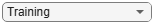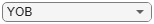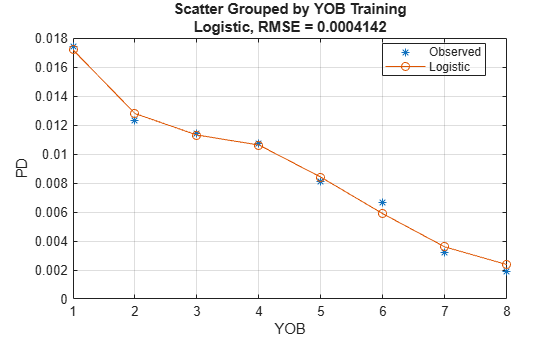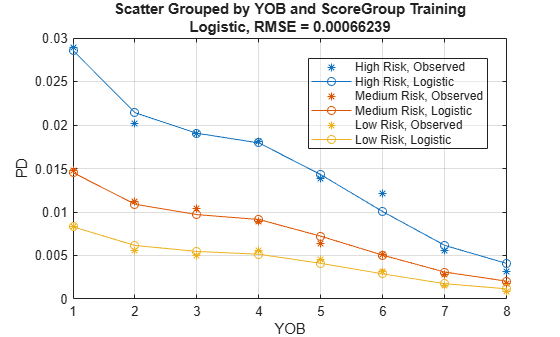# modelAccuracy

Compute RMSE of predicted and observed PDs on grouped data

## Syntax

``AccMeasure = modelAccuracy(pdModel,data,GroupBy)``
``[AccMeasure,AccData] = modelAccuracy(___,Name,Value)``

## Description

example

````AccMeasure = modelAccuracy(pdModel,data,GroupBy)` computes the root mean squared error (RMSE) of the observed compared to the predicted probabilities of default (PD). `GroupBy` is required and can be any column in the `data` input (not necessarily a model variable). The `modelAccuracy` function computes the observed PD as the default rate of each group and the predicted PD as the average PD for each group. `modelAccuracy` supports comparison against a reference model.```

example

````[AccMeasure,AccData] = modelAccuracy(___,Name,Value)` specifies options using one or more name-value pair arguments in addition to the input arguments in the previous syntax.```

## Examples

collapse all

This example shows how to use `fitLifetimePDModel` to fit data with a `Logistic` model and then use `modelAccuracy` to compute the root mean squared error (RMSE) of the observed probabilities of default (PDs) with respect to the predicted PDs.

```load RetailCreditPanelData.mat disp(head(data))```
``` ID ScoreGroup YOB Default Year __ __________ ___ _______ ____ 1 Low Risk 1 0 1997 1 Low Risk 2 0 1998 1 Low Risk 3 0 1999 1 Low Risk 4 0 2000 1 Low Risk 5 0 2001 1 Low Risk 6 0 2002 1 Low Risk 7 0 2003 1 Low Risk 8 0 2004 ```
`disp(head(dataMacro))`
``` Year GDP Market ____ _____ ______ 1997 2.72 7.61 1998 3.57 26.24 1999 2.86 18.1 2000 2.43 3.19 2001 1.26 -10.51 2002 -0.59 -22.95 2003 0.63 2.78 2004 1.85 9.48 ```

Join the two data components into a single data set.

```data = join(data,dataMacro); disp(head(data))```
``` ID ScoreGroup YOB Default Year GDP Market __ __________ ___ _______ ____ _____ ______ 1 Low Risk 1 0 1997 2.72 7.61 1 Low Risk 2 0 1998 3.57 26.24 1 Low Risk 3 0 1999 2.86 18.1 1 Low Risk 4 0 2000 2.43 3.19 1 Low Risk 5 0 2001 1.26 -10.51 1 Low Risk 6 0 2002 -0.59 -22.95 1 Low Risk 7 0 2003 0.63 2.78 1 Low Risk 8 0 2004 1.85 9.48 ```

Partition Data

Separate the data into training and test partitions.

```nIDs = max(data.ID); uniqueIDs = unique(data.ID); rng('default'); % For reproducibility c = cvpartition(nIDs,'HoldOut',0.4); TrainIDInd = training(c); TestIDInd = test(c); TrainDataInd = ismember(data.ID,uniqueIDs(TrainIDInd)); TestDataInd = ismember(data.ID,uniqueIDs(TestIDInd));```

Create `Logistic` Lifetime PD Model

Use `fitLifetimePDModel` to create a `Logistic` model using the training data.

```pdModel = fitLifetimePDModel(data(TrainDataInd,:),"Logistic",... 'AgeVar','YOB',... 'IDVar','ID',... 'LoanVars','ScoreGroup',... 'MacroVars',{'GDP','Market'},... 'ResponseVar','Default'); disp(pdModel)```
``` Logistic with properties: ModelID: "Logistic" Description: "" Model: [1x1 classreg.regr.CompactGeneralizedLinearModel] IDVar: "ID" AgeVar: "YOB" LoanVars: "ScoreGroup" MacroVars: ["GDP" "Market"] ResponseVar: "Default" ```

Display the underlying model.

`disp(pdModel.Model)`
```Compact generalized linear regression model: logit(Default) ~ 1 + ScoreGroup + YOB + GDP + Market Distribution = Binomial Estimated Coefficients: Estimate SE tStat pValue __________ _________ _______ ___________ (Intercept) -2.7422 0.10136 -27.054 3.408e-161 ScoreGroup_Medium Risk -0.68968 0.037286 -18.497 2.1894e-76 ScoreGroup_Low Risk -1.2587 0.045451 -27.693 8.4736e-169 YOB -0.30894 0.013587 -22.738 1.8738e-114 GDP -0.11111 0.039673 -2.8006 0.0051008 Market -0.0083659 0.0028358 -2.9502 0.0031761 388097 observations, 388091 error degrees of freedom Dispersion: 1 Chi^2-statistic vs. constant model: 1.85e+03, p-value = 0 ```

Compute Model Accuracy

Model accuracy measures how accurate the predicted probabilities of default are. For example, if the model predicts a 10% PD for a group, does the group end up showing an approximate 10% default rate, or is the eventual rate much higher or lower? While model discrimination measures the risk ranking only, model accuracy measures the accuracy of the predicted risk levels.

`modelAccuracy` computes the root mean squared error (RMSE) of the observed PDs with respect to the predicted PDs. A grouping variable is required and it can be any column in the data input (not necessarily a model variable). The `modelAccuracy` function computes the observed PD as the default rate of each group and the predicted PD as the average PD for each group.

```DataSetChoice ="Training"; if DataSetChoice=="Training" Ind = TrainDataInd; else Ind = TestDataInd; end GroupingVar ="YOB"; AccMeasure = modelAccuracy(pdModel,data(Ind,:),GroupingVar,'DataID',DataSetChoice); disp(AccMeasure) ```
``` RMSE _________ Logistic, grouped by YOB, Training 0.0004142 ```

Visualize the model accuracy using `modelAccuracyPlot`.

`modelAccuracyPlot(pdModel,data(Ind,:),GroupingVar,'DataID',DataSetChoice);`You can use more than one variable for grouping. For this example, group by the variables `YOB` and `ScoreGroup`.

```AccMeasure = modelAccuracy(pdModel,data(Ind,:),["YOB","ScoreGroup"],'DataID',DataSetChoice); disp(AccMeasure)```
``` RMSE __________ Logistic, grouped by YOB, ScoreGroup, Training 0.00066239 ```

Now visualize the two grouping variables using using `modelAccuracyPlot`.

`modelAccuracyPlot(pdModel,data(Ind,:),["YOB","ScoreGroup"],'DataID',DataSetChoice);`## Input Arguments

collapse all

Probability of default model, specified as a `Logistic` or `Probit` object previously created using `fitLifetimePDModel`.

Note

The `'ModelID'` property of the `pdModel` object is used as the identifier or tag for `pdModel`.

Data Types: `object`

Data, specified as a `NumRows`-by-`NumCols` table with projected predictor values to make lifetime predictions. The predictor names and data types must be consistent with the underlying model.

Data Types: `table`

Name of column in the `data` input used to group the data, specified as a string or character vector. `GroupBy` does not have to be a model variable name. For each group designated by `GroupBy`, the `modelAccuracy` function computes the observed default rates and average predicted PDs are computed to measure the RMSE.

Data Types: `string` | `char`

### Name-Value Pair Arguments

Specify optional comma-separated pairs of `Name,Value` arguments. `Name` is the argument name and `Value` is the corresponding value. `Name` must appear inside quotes. You can specify several name and value pair arguments in any order as `Name1,Value1,...,NameN,ValueN`.

Example: ```[AccMeasure,AccData] = modelAccuracy(pdModel,data(Ind,:),'GroupBy',["YOB","ScoreGroup"],'DataID',DataSetChoice)```

Data set identifier, specified as the comma-separated pair consisting of `'DataID'` and a character vector or string. `DataID` is included in the `modelAccuracy` output for reporting purposes.

Data Types: `char` | `string`

Conditional PD values predicted for `data` by the reference model, specified as the comma-separated pair consisting of `'ReferencePD'` and a `NumRows`-by-`1` numeric vector. The functions reports the `modelAccuracy` output information for both the `pdModel` object and the reference model.

Data Types: `double`

Identifier for the reference model, specified as the comma-separated pair consisting of `'ReferenceID'` and a character vector or string. `ReferenceID` is used in the `modelAccuracy` output for reporting purposes.

Data Types: `char` | `string`

## Output Arguments

collapse all

Accuracy measure, returned as a table.

RMSE values, returned as a single-column `'RMSE'` table. The table has one row if only the `pdModel` accuracy is measured and it has two rows if reference model information is given. The row names of `AccMeasure` report the model IDs, grouping variables, and data ID.

Note

The reported RMSE values depend on the grouping variable for the required `GroupBy` argument.

Accuracy data, returned as a table.

Observed and predicted PD values for each group, returned as a table. The reported observed PD values correspond to the observed default rate for each group. The reported predicted PD values are the average PD values predicted by the `pdModel` object for each group, and similarly for the reference model. The `modelAccuracy` function stacks the PD data, placing the observed values for all groups first, then the predicted PDs for the `pdModel`, and then the predicted PDs for the reference model, if given.

The column `'ModelID'` identifies which rows correspond to the observed PD, `pdModel`, or reference model. The table also has one column for each grouping variable showing the unique combinations of grouping values. The last column of `AccData` is a `'PD'` column with the PD data.

collapse all

### Model Accuracy

Model accuracy measures the accuracy of the predicted probability of default (PD) values.

To measure model accuracy, also called model calibration, you must compare the predicted PD values to the observed default rates. For example, if a group of customers is predicted to have an average PD of 5%, then is the observed default rate for that group close to 5%?

The `modelAccuracy` function requires a grouping variable to compute average predicted PD values within each group and the average observed default rate also within each group. `modelAccuracy` uses the root mean squared error (RMSE) to measure the deviations between the observed and predicted values across groups. For example, the grouping variable could be the calendar year, so that rows corresponding to the same calendar year are grouped together. Then, for each year the software computes the observed default rate and the average predicted PD. The `modelAccuracy` function then applies the RMSE formula to obtain a single measure of the prediction error across all years in the sample.

Suppose there are N observations in the data set, and there are M groups G1,…,GM. The default rate for group Gi is

`$D{R}_{i}=\frac{{D}_{i}}{{N}_{i}}$`

where:

Di is the number of defaults observed in group Gi.

Ni is the number of observations in group Gi.

The average predicted probability of default PDi for group Gi is

`$P{D}_{i}=\frac{1}{{N}_{i}}{\sum }_{j\in {G}_{i}}PD\left(j\right)$`

where PD(j) is the probability of default for observation j. In other words, this is the average of the predicted PDs within group Gi.

Therefore, the RMSE is computed as

`$RMSE\text{​}=\sqrt{{\sum }_{i=1}^{M}\left(\frac{{N}_{i}}{N}\right){\left(D{R}_{i}-P{D}_{i}\right)}^{2}}$`

The RMSE, as defined, depends on the selected grouping variable. For example, grouping by calendar year and grouping by years-on-books might result in different RSME values.

Use `modelAccuracyPlot` to visualize observed default rates and predicted PD values on grouped data.

 Baesens, Bart, Daniel Roesch, and Harald Scheule. Credit Risk Analytics: Measurement Techniques, Applications, and Examples in SAS. Wiley, 2016.

 Bellini, Tiziano. IFRS 9 and CECL Credit Risk Modelling and Validation: A Practical Guide with Examples Worked in R and SAS. San Diego, CA: Elsevier, 2019.

 Breeden, Joseph. Living with CECL: The Modeling Dictionary. Santa Fe, NM: Prescient Models LLC, 2018.